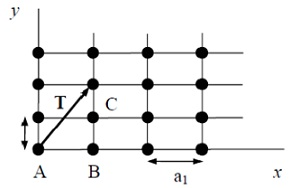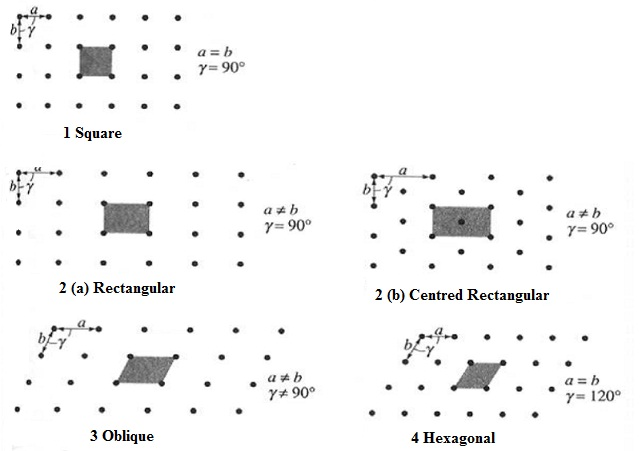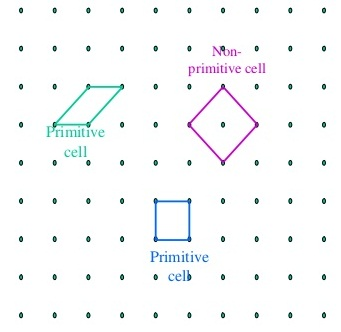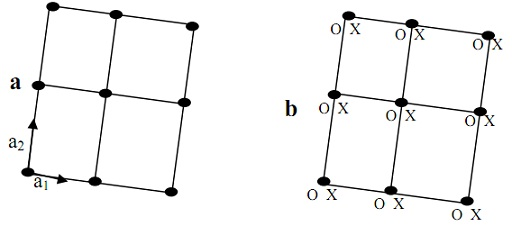#### Crystal geometry, Physics tutorial

Introduction:

The physical statement or definition of a solid consists of some ingredients. We began by defining a solid as a large collection of atoms which attract one another so as to confine the atoms to a definite volume of space. The crystalline solid is a material whose atoms encompass a regular arrangement which show translational symmetry. Whenever we state that the atoms have a regular arrangement, what we signify is that the equilibrium positions of the atoms encompass a regular arrangement. At any specific temperature, the atoms might vibrate having small amplitudes regarding fixed equilibrium positions. The elements form solids because for some range of temperature and pressure, a solid consists of less free energy than other states of matter. This is usually assumed that at low adequate temperature and by means of appropriate external pressure everything becomes a solid. The study of crystal and electrons in crystal is a division of physics termed as solid state physics. The solid state physics is the extension of atomic physics following the discovery of X-ray diffractions of the crystalline properties.

Definition of crystal:

Crystal might state on the macroscopic scale as homogeneous solids, in which a few of the physical properties are function of direction. Microspically, a crystal might be stated as a solid having an arrangement of atoms (or molecules) in which the atoms are set in some repetitive pattern in (3-D) three dimensions.

Translational Symmetry:

A solid is stated to be a crystal if atoms are ordered in such a manner that their positions are precisely periodic. This theory is described in the figure below by using a two-dimensional (2-D) structure. A perfect crystal maintains this periodicity in both the x and y directions from - ∞ to + ∞. As pursues from this periodicity, the atoms A, B, C and so on are equivalent. In another words, for an observer positioned at any of such atomic sites, the crystal appears precisely the same. The similar idea can be deduced by saying that a crystal encompasses a translational symmetry. The translational symmetry signifies that if the crystal is translated via any vector joining two atoms, says 'T' in the figure shown below, the crystal appears precisely the same as it did before the translation. In another words the crystal remains invariant beneath any such translation.Lattice and Unit cell:

The structure of each and every crystal can be illustrated in terms of a lattice. A lattice can be stated as a regular periodic array of points in space. Each and every lattice point can be positioned as:

rmn = ma + nb

Or in three dimensional (3-D) cases:

rlmn = la + mb + nc

Here a, b, c is termed as Lattice vectors and l, m and n are integers.

The network of lattice lines split or divide the space into similar parts termed as unit cells. Therefore, because of inherent periodicity of space lattice; it can therefore be represented through a unit cell. A unit cell is a suitably chosen basic block by repeating the whole space lattice which is produced. The unit cell might be in form of a parallelogram (2D) or a parallelepiped (3D) having lattice points at their corners. The shape and size of the unit cell are illustrated by three lattice vectors a, b, c, originating from one corner of the unit cell. The axial lengths a, b, c and the inter axial angles α, β and γ are lattice parameters of the unit cell. The figure shown below represents the unit cell having the axes lengths and inter axial angles whereas the next figure represents the lattice and unit cells in 2-dimension.The convention for sketching the lattice parameters is as shown below:

a: parallel to x-axis

b: parallel to y-axis

c: parallel to z-axis

α: angle between y and z

β: angle between z and x

γ: angle between x and yPrimitive and Non-Primitive cells:

The cell is stated to be primitive if the lattice points  are at the corners of the cell as shown in figure below and if there are lattice points in the cell other than the corners, then the cell is stated to be non-primitive as shown in figure below.For a single atom, the single atom is positioned on the lattice site and is termed as Bravais lattice. On the other hand, if there are some atoms per unit cell, we include a lattice with a basis.

Bravais Lattice:

There are numerous ways in which an actual crystal might be built, therefore possible crystal structures are unlimited. Though, the possible schemes of space lattices are highly restricted. Each and every space lattice consists of some convenient set of axes that need not be essentially orthogonal and chosen length all along the three axes might not be equivalent. Bravais in the year 1848 proved that there are only fourteen space lattices in total that are needed to illustrate all the possible arrangement of points in space subject to the condition that each and every lattice point has precisely similar environment. The fourteen space environments are termed as Bravais Lattices. The Bravais lattices are the distinct lattice types which whenever repeated can fill the entire space. The lattice can thus be produced by three unit vectors, a, b and c and a set of integers k, l and m so that each and every lattice point, recognized by a vector r, can be obtained from:

r = k a + l b + m c

Bravais represented that in two dimensions there are five dissimilar Bravais lattices, whereas in three dimensions there exist no more than fourteen space lattices.

Basis and Crystal structure:

The arrangement of atoms in the solid is known as crystal structure. In order to change or transform the geometrical array of points in space (that is, lattice) into a crystal structure, we should locate atoms or molecules on the lattice points. The repeating unit assembly of atoms or molecules which are positioned at each and every lattice point is termed as the basis. The basis should be similar in composition, arrangement and orientation in such a way that the crystal appears precisely similar at one point as it doesn't at other equivalent points. No basis includes fewer atoms than a primitive basis contains.

The crystal structure is therefore given by two specifications:

1) The lattice

2) The assembly that repeat itself

Therefore, the logical relation is:

Space lattice + basis = crystal structureTutorsglobe: A way to secure high grade in your curriculum (Online Tutoring)

Expand your confidence, grow study skills and improve your grades.

Since 2009, Tutorsglobe has proactively helped millions of students to get better grades in school, college or university and score well in competitive tests with live, one-on-one online tutoring.

Using an advanced developed tutoring system providing little or no wait time, the students are connected on-demand with a tutor at www.tutorsglobe.com. Students work one-on-one, in real-time with a tutor, communicating and studying using a virtual whiteboard technology.  Scientific and mathematical notation, symbols, geometric figures, graphing and freehand drawing can be rendered quickly and easily in the advanced whiteboard.

Free to know our price and packages for online physics tutoring. Chat with us or submit request at info@tutorsglobe.com